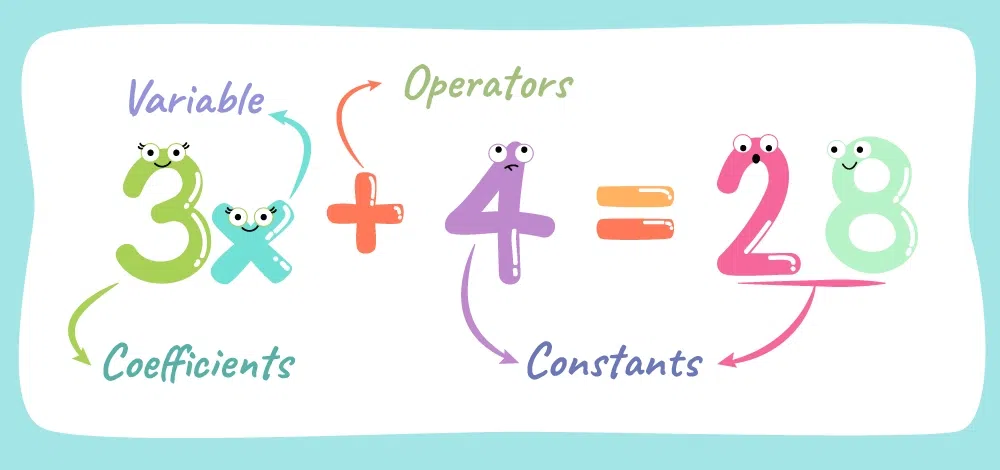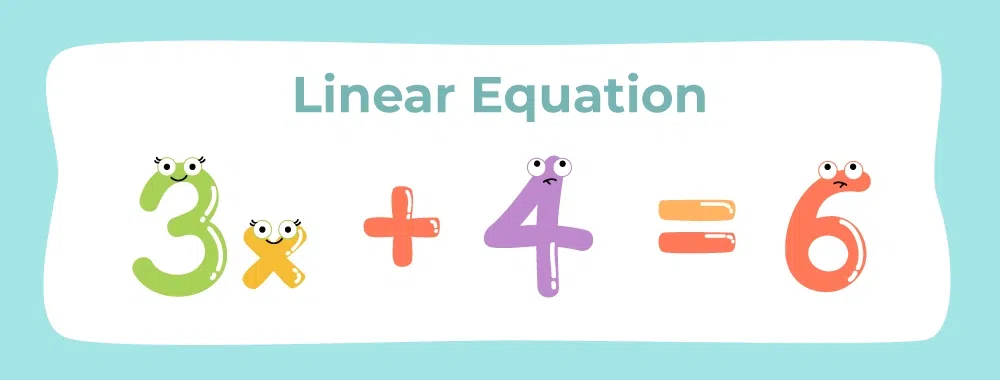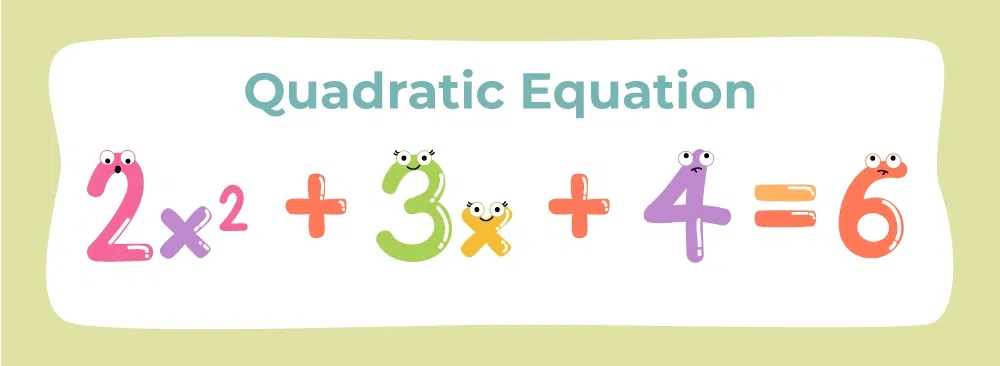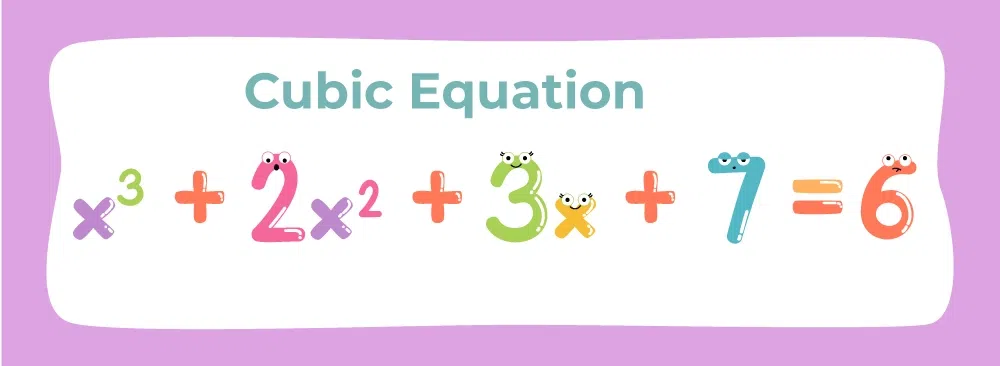GeeksforGeeks App
Open AppBrowser
Continue

# Algebra

Algebra is the mathematical study of number theory, geometry, and analysis. It is one of the oldest fields of mathematics. According to some definitions, algebra is the study of mathematical rules and symbols as well as changes in these mathematical symbols. Algebra includes a wide range of topics, including observing abstractions and solving simple mathematical puzzles. Several chapters of the mathematics textbooks that pupils will read in school contain algebraic equations. We have algebraic identities and algebraic formulae to solve algebraic expressions.

## What is Algebra?

Algebra mainly focuses on variables, which have unknown values. These variables may change. Variables are symbols like x, y, z, p, or q. Mathematical operations like addition, subtraction, multiplication, and division are combined with variables like x, y, and z to form a meaningful equation. It follows a set of laws when performing mathematical operations. When analyzing data sets with two or more variables, the rules are used. Linear algebra, advanced algebra, abstract algebra, basic algebra, and commutative algebra are some branches of algebra.

## Branches of Algebra

The usage of several Algebraic expressions reduces the complexity of Algebra. The various branches of Algebra based on the use and complexity of the expressions are as such:

1. Pre Algebra
2. Elementary Algebra
3. Abstract Algebra
4. Universal Algebra
5. Linear Algebra
6. Commutative Algebra

### Pre Algebra

It includes the fundamental concepts of arithmetic and Algebra, such as order of operations, basic operations with numbers, and simplifying expressions. It assists in turning day-to-day problems into mathematical expressions that use Algebra. Pre-Algebra includes creating a mathematical expression for the provided problem statement.

### Elementary Algebra

The goal of elementary Algebra is to find a solution by resolving Algebraic expressions. Simple variables like x and y are expressed as equations in elementary Algebra. The equations are divided into polynomials, quadratic equations, or linear equations depending on the degree of the variable. The formulas for linear equations are ax + b = c, ax+ by + c = 0, and ax + by + cz + d = 0. Based on the number of variables, quadratic equations, and polynomials are subsets of Elementary Algebra. For a polynomial problem, the typical form of representation is axn + bxn-1+ cxn-2+…..k = 0, while for a quadratic equation, it is ax2 + bx + c = 0.

### Abstract Algebra

It is a branch of mathematics that focuses on Algebraic systems like groups, rings, fields, and modules, rather than on specific numerical computations. In abstract Algebra, we do not study specific operations like addition and multiplication but instead study general properties of basic operations, such as associativity, commutativity, distributivity, and the existence of inverses.  Groups, sets, modules, rings, lattices, vector spaces, and other Algebraic structures are studied in abstract Algebra.

### Universal Algebra

Universal Algebra can be used to explain all other mathematical forms using Algebraic expressions in coordinate geometry, calculus, and trigonometry. In each of these areas, universal Algebra focuses on equations rather than Algebraic models. You can think of all other types of Algebra as being a subset of universal Algebra. Any real-world issue can be categorized into a particular discipline of mathematics and solved using abstract Algebra.

### Linear Algebra

Linear algebra, a branch of algebra, finds uses in both pure and practical mathematics. It deals with the linear mappings of the vector spaces. It also involves learning about lines and planes. It is the study of linear systems of equations with transformational features. It is used in almost all areas of mathematics. It deals with the representation of linear equations for linear functions in matrices and vector spaces.

### Commutative Algebra

Commutative algebra is one of the types of algebra that studies commutative rings and their ideals. Both algebraic geometry and algebraic number theory require commutative algebra. Rings of algebraic integers, polynomial rings, and other rings are all present. Numerous other areas of mathematics, such as differential topology, invariant theory, order theory, and generic topology, make use of commutative algebra.

## Algebra Topics

Algebra is divided into a number of concepts to help in our understanding of it. The key algebraic ideas included here are sets, exponents, logarithms, series, and sequences, as well as algebraic expressions and equations.

### Algebraic Expressions

Algebraic Expressions consist of constants and variables. We can add, subtract, multiply, and divide these expressions. An example of an algebraic expression is 5x + 6. The variables might also have values like x2, x3, xn, xy, x2y, etc. Polynomials is also a term used for algebraic expressions. An equation that contains coefficients, non-negative integer exponents of variables, and variables is called a polynomial. For example, 5x3 + 4x2 + 7x + 2 = 0.An algebraic equation shows the connection between two quantities when one or both of the values are unknown.

Given below are the different types of Algebraic equations based on the degree of the variable:

1. Linear Equations
3. Cubic Equations

### Linear Equations

Linear equation is an equation in which the highest power of a variable is 1. They are also known as first-order equations. A linear equation is an equation for a straight line when seen in the coordinate system. The straight-line equation is written as y = mx + b, where m denotes the line’s slope and b denotes the y-intercept.Following are the topics that discuss linear equations deeply:

A quadratic equation is a type of Algebraic equation that contains one or more terms in which the variable is raised to the power of 2 (i.e., a quadratic term). It is an equation of the form ax2 + bx + c = 0, where a, b, and c are constants and x is the variable.Here are the topics that discuss quadratic equations thoroughly.

### Cubic Equations

A three-degree equation, or a cubic equation, has a variable whose maximum power is 3. A cubic equation has the general form ax3 + bx2 + cx + d = 0.

where x is a variable and a, b, c, and d are constants. A cubic equation can have one, two, or three solutions that are real or complex numbers, depending on the coefficients in the equation.### Polynomials

Polynomials are made by variables and coefficients, which are Algebraic expressions. Indeterminate is another name for variables. For polynomials, we can perform addition, subtraction, multiplication, and positive integer exponents, but not division by variable. For ex: 3x3-5x+8 .

Following are the topics that discuss polynomials deeply:

### Sequence and Series

An ordered collection of numbers or other elements of mathematics that follow a pattern or rule is called a sequence. The position of each term of a sequence within the sequence is marked by its index or subscript. The series of even numbers, for example, can be written as 2, 4, 6, 8, 10, and so on.

The total of a sequence’s terms forms a series. For instance, the series 2 + 4 + 6 +… + 2n gives the sum of the first n terms of the even number sequence. Series may be finite or infinite.

Sequence and Series can be classified into two major categories – Arithmetic Progression and Geometric Progression.

### Arithmetic Progression

Arithmetic Progression(A.P) is a series of numbers where each term is obtained by adding a constant /fixed value to the previous term. This constant difference in the terms is denoted by ‘d’. The general format of an A.P is:

a, a + d, a + 2d, a + 3d, …, a + nd

where a is the first term, d is a common difference, and n is the number of terms.

### Geometric Progression

Geometric Progression(G.P) is a series of numbers where each term is obtained by multiplying the preceding term by a fixed constant value called the common ratio, denoted by r.

The general form of a geometric progression is:

a, ar, ar2, ar3, …, arn

where a is the first term, r is the common ratio, and n is the number of terms.

Given is the list of topics that will give you a better understanding of sequence and series.

### Exponents

Exponent is a mathematical operation, written as an where a is the base and n is the power or the exponent. Exponents help us simplify Algebraic expressions. Exponent can be represented in the form

an = a*a*a*….n times.

### Logarithms

The Algebraic opposite of exponents is the logarithm. It is practical to simplify complicated Algebraic formulas using logarithms. The exponential form denoted by the formula ax = n can be converted to logarithmic form by using the formula log a n = x.

In 1614, John Napier discovered logarithms. Nowadays, logarithms are a crucial component of modern mathematics.

### Set Theory

Set theory is a branch of mathematical logic that investigates sets, which are arrays of objects informally. The term “set” refers to a well-defined group of unique items that are used to express Algebraic variables. Sets are used to depict the collection of important elements in a group. Sets can be expressed in set-builder or roster form. Sets are usually denoted by curly braces;{} for example, A = {1,2,3,4} is a collection. Let’s learn more about the sets in the following articles:

### Vectors

One of the most important aspects of Algebra is vector Algebra. It is a course that focuses on the Algebra of vector quantities. As we all know, there are two kinds of measurable quantities: scalars and vectors. The magnitude of a scalar quantity is the only thing that matters, while the magnitude and direction of a vector quantity are also essential. A vector is a two-dimensional object of both magnitude and direction. It is normally represented by an arrow with a length (→) that indicates the magnitude and direction. It is denoted by the letter V. The vector’s magnitude is denoted by the letter |V|. Let’s discuss the vector and its Algebra in the following articles:

### Relations and Functions

The most significant subjects in mathematics are “Relations and Functions.” Relations and functions are two distinct terms that have different mathematical interpretations. One might be puzzled by the differences between them. Before we go even further, let’s look at a clear example of the differences between the two. An ordered pair is represented as (INPUT, OUTPUT): The relation shows the relationship between INPUT and OUTPUT. Whereas, a function is a relation that derives one OUTPUT for each given INPUT. Let’s discuss more of the topic in the following articles:

### Matrices and Determinants

In linear Algebra, determinants, and matrices are used to solve linear equations by applying Cramer’s law to a series of non-homogeneous linear equations. Only square matrices are used to measure determinants. While a matrix’s determinant is empty, it’s known as a singular determinant, and when its determinant is one, it’s known as unimodular. The determinant of the matrix must be nonsingular, that is, its value must be nonzero, for the set of equations to have a unique solution. Let us look at the definitions of determinants and matrices, as well as the various forms of matrices and their properties, using examples in the following articles:

### Permutations and Combinations

Permutation and Combination are methods for representing a collection of objects by choosing them from a list and dividing them into subsets. It specifies the different methods for organizing a set of data. Permutations are used to choose data or events from a group, while combinations are used to represent the order in which they are represented. Let’s discuss them deeply in the following articles:

### Mathematical Induction

For every natural number n, mathematical induction is a technique for proving a proposition, hypothesis, or formula that is assumed to be valid. The ‘Principle of Mathematical Induction is a generalization of this that we can use to prove any mathematical statement. Therefore, let’s learn more about it in the following articles:

## Conclusion

In this article, we learned different concepts of Algebra and the applications of Algebra which will help students to have a better understanding of the principles of Algebraic equations and how to solve them and implement them to solve real-world problems.

## Problems on Algebra

Problem 1: Solve  3ex + 6 = 120

Solution:

Given,

3ex + 6 = 120

3ex = 120 – 6

3ex = 114

ex = 114/3

ex = 38

x = ln 38

Problem 2: Solve for the value of y : 2(4-y)-3(y+3)=11

Solution:

2(4-y) -3(y+3)=11

8-2y-3y-9=11

-5y-1=-11

-5y-1+1=-11+1

-5y=-10

Dividing both the sides by -5,

-5y/-5=-10/-5

y=2

Problem 3: Evaluate (2.3a5b2) × (1.2a2b2) when a = 1 and b = 0.5

Solution:

Let us simplify the given expression

=2.3a5b2 × 1.2a2b2

=2.3 × 1.2 × a5 × a2 × b2 × b2

=2.76 × a5+2 × b2+2

=2.76a7b4

Now let us substitute when, a = 1 and b = 0.5

For 2.76 a7 b4

=2.76 (1)7 (0.5)4

=2.76 × 1 × 0.0025

=0.1725

=6.9/40

Problem 4: (2x-1)/3 – (6x-2)/5 = 1/3

Solution:

We have,

(2x-1)/3 – (6x-2)/5 = 1/3

By taking LCM for 3 and 5, which is 15

((2x-1)×5)/15 – ((6x-2)×3)/15 = 1/3

(10x – 5)/15 – (18x – 6)/15 = 1/3

(10x – 5 – 18x + 6)/15 = 1/3

(-8x + 1)/15 = 1/3

By using cross-multiplication, we get,

(-8x + 1)3 = 15

-24x + 3 = 15

-24x = 15 – 3

-24x = 12

x = -12/24

= -1/2

∴ x = -1/2

Verification

LHS = (2x – 1)/3 – (6x – 2)/5

= [2(-1/2) – 1]/3 – [6(-1/2) – 2]/5

= (- 1 – 1)/3 – (-3 – 2)/5

= – 2/3 – (-5/5)

= -2/3 + 1

= (-2 + 3)/3

= 1/3

RHS

Problem 5: Find a number such that when 5 is subtracted from 5 times that number, the result is 4, more than twice the number.

Solution:

Let us consider the number as ‘x’

Then, five times the number will be 5x

And, two times, the number will be 2x

So,

5x – 5 = 2x + 4

5x – 2x = 5 + 4

3x = 9

x = 9/3

x = 3

∴ The number is 3.

## FAQs on Algebra

### Q1. What is Algebra?

Algebra is the area of mathematics that deals with the representation of mathematical statements as solutions to problems. To create a meaningful mathematical expression, it takes variables like x, y, and z together with mathematical operations like addition, subtraction, multiplication, and division.

### Q2. What are the branches of Algebra?

The branches of Algebra are –

1. Pre Algebra
2. Elementary Algebra
3. Abstract Algebra
4. Universal Algebra
5. Linear Algebra
6. Commutative Algebra

### Q3. Why students are required to learn Algebra?

Algebra is important for students to study because it develops problem-solving abilities, prepares them for higher-level arithmetic, helps in understanding and analyzing real-world problems, and has applications in a wide range of professions, including science, engineering, economics, and finance. A lot of college majors and jobs also require Algebra.